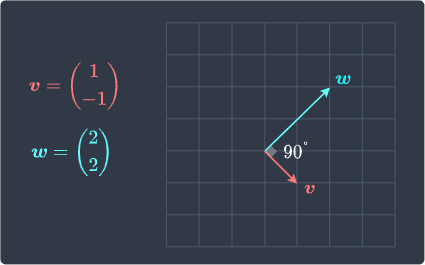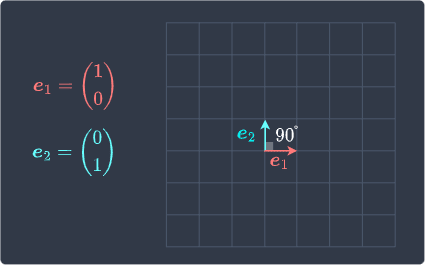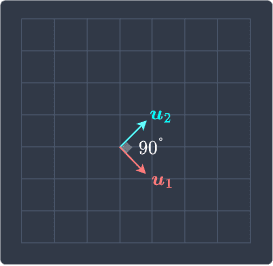search
Search
Unlock 100+ guides
search toc
close
account_circle
Profile
exit_to_app
Sign out
search
keyboard_voice
close
Searching Tips
Search for a recipe:
"Creating a table in MySQL"
Search for an API documentation: "@append"
Search for code: "!dataframe"
Apply a tag filter: "#python"
Useful Shortcuts
/ to open search panel
Esc to close search panel
to navigate between search results
d to clear all current filters
Enter to expand content previewDoc SearchCode Search BetaSORRY NOTHING FOUND!
mic
Start speaking...Voice search is only supported in Safari and Chrome.
Shrink
Navigate to

# Guide on Orthonormal Sets and Bases

schedule Aug 12, 2023
Last updated
local_offer
Linear Algebra
Tags
Definition.

# Orthogonal sets

A set of two or more vectors is said to be orthogonal if every pair of vectors in the set is orthogonal.

Example.

## Showing that a set is orthogonal

Consider the following set of vectors:

$$\boldsymbol{v}= \begin{pmatrix} 1\\-1 \end{pmatrix},\;\;\;\;\; \boldsymbol{w}= \begin{pmatrix}2\\2 \end{pmatrix}$$

Show that these vectors form an orthogonal set.

Solution. To show that vectors $\boldsymbol{v}$ and $\boldsymbol{w}$ form an orthogonal set, we must check that they are perpendicular to each other. We know from theoremlink that if the dot product of two vectors is zero, then they are perpendicular. So let's compute the dot product between them:

\begin{align*} \begin{pmatrix} 1\\-1 \end{pmatrix}\cdot \begin{pmatrix} 2\\2 \end{pmatrix}&= (1)(2)+(-1)(2)\\&=0 \end{align*}

Because $\boldsymbol{v}$ and $\boldsymbol{w}$ are orthogonal to each other, we conclude that they form an orthogonal set. Let's also visually confirm this:Indeed, they are perpendicular!

Definition.

# Orthonormal set

An orthonormal set is an orthogonal set of unit vectors. In other words, a set of vectors is orthonormal if and only if:

• every pair of vectors is perpendicular.

• every vector has a length of one.

Example.

## Showing that a set is orthonormal

Consider the standard basis vectors for $\mathbb{R}^2$ below:

$$\boldsymbol{e}_1= \begin{pmatrix} 1\\0 \end{pmatrix},\;\;\;\;\; \boldsymbol{e}_2= \begin{pmatrix}0\\1 \end{pmatrix}$$

Show that these vectors form an orthonormal set.

Solution. Let's first check that they are perpendicular to each other:

\begin{align*} \boldsymbol{e}_1\cdot\boldsymbol{e}_2&= \begin{pmatrix} 1\\0 \end{pmatrix}\cdot \begin{pmatrix} 0\\1 \end{pmatrix}\\ &=(1)(0)+(0)(1)\\ &=0 \end{align*}

Because their dot product is zero, they are perpendicular. We can also easily tell that these vectors have both length one, and so $\boldsymbol{e}_1$ and $\boldsymbol{e}_2$ form an orthonormal set by definition!

We can visually confirm this as well:Theorem.

# Constructing an orthonormal set from an orthogonal set

Given an orthogonal set, we can construct an orthonormal set by normalizing each vector $\boldsymbol{v}$ to a unit vector $\boldsymbol{u}$ like so:

$$\begin{equation}\label{eq:WDgE7iuxsGj6txyl1ss} \boldsymbol{u}= \frac{1}{\Vert{\boldsymbol{v}}\Vert}\boldsymbol{v} \end{equation}$$

Where $\Vert\boldsymbol{v}\Vert$ is the magnitudelink of $\boldsymbol{v}$.

Proof. We know from theoremlink that:

• the vector $\boldsymbol{u}$ in formula \eqref{eq:WDgE7iuxsGj6txyl1ss} has a length of one.

• the vector $\boldsymbol{u}$ points in the same direction as $\boldsymbol{v}$.

Since the direction of every vector in the orthogonal set is preserved, the new unit vectors will also be perpendicular to each other. This means that the new set of vectors is orthonormal. This completes the proof.

Example.

## Constructing an orthonormal set using an orthogonal set

Consider the following orthogonal set of vectors:

$$\boldsymbol{v}= \begin{pmatrix} 1\\-1 \end{pmatrix},\;\;\;\;\; \boldsymbol{w}= \begin{pmatrix}2\\2 \end{pmatrix}$$

Construct an orthonormal set.

Solution. To convert an orthogonal set of vectors into an orthonormal set, we simply have to convert them into unit vectors. Let's convert $\boldsymbol{v}$ into a unit vector first:

\begin{align*} \boldsymbol{u}_1&= \frac{1}{\Vert{\boldsymbol{v}}\Vert}\boldsymbol{v}\\ &=\frac{1}{\big((1)^2+(-1)^2\big)^{1/2}} \begin{pmatrix} 1\\-1 \end{pmatrix}\\ &=\frac{1}{\sqrt{2}}\begin{pmatrix} 1\\-1 \end{pmatrix} \end{align*}

Next, let's convert $\boldsymbol{w}$ into a unit vector:

\begin{align*} \boldsymbol{u}_2&= \frac{1}{\Vert{\boldsymbol{w}}\Vert}\boldsymbol{w}\\ &=\frac{1}{\big((2)^2+(2)^2\big)^{1/2}} \begin{pmatrix} 2\\2 \end{pmatrix}\\ &=\frac{1}{2\sqrt{2}}\begin{pmatrix} 2\\2 \end{pmatrix} \end{align*}

Visually, $\boldsymbol{u}_1$ and $\boldsymbol{u}_2$ look as follows:Theorem.

# Orthogonal sets are linearly independent

If $S$ is an orthogonal set of non-zero vectors, then $S$ is linearly independent. This means that orthonormal sets, which are special cases of orthogonal sets, are also linearly independent.

Proof. Suppose $S$ is an orthogonal set with the following vectors:

$$S=\{ \boldsymbol{v}_1, \boldsymbol{v}_2,\cdots \boldsymbol{v}_n \}$$

We can use the definitionlink of linear independence to show that $S$ is linearly independent:

$$\begin{equation}\label{eq:bdBBKeLSAfSp81Aw4Eb} c_1\boldsymbol{v}_1+ c_2\boldsymbol{v}_2+ \cdots+ c_n\boldsymbol{v}_n= \boldsymbol{0} \end{equation}$$

If the only way for this equality to hold is $c_1=c_2=\cdots=c_n$, then $S$ is linearly independent.

Let's perform a dot product of both sides of \eqref{eq:bdBBKeLSAfSp81Aw4Eb} with $\boldsymbol{v}_i$ where $i$ is any value between $1$ and $n$ to get:

$$\begin{equation}\label{eq:eKibp1fyLn3VM48eUi7} c_1\boldsymbol{v}_1\cdot\boldsymbol{v}_i+ c_2\boldsymbol{v}_2\cdot\boldsymbol{v}_i+ \cdots+ c_n\boldsymbol{v}_n\cdot\boldsymbol{v}_i= 0 \end{equation}$$

Because every pair of vectors in $S$ is orthogonal, we have that $\boldsymbol{v}_j\cdot{\boldsymbol{v}_i}=0$ if $j\ne{i}$. Therefore, \eqref{eq:eKibp1fyLn3VM48eUi7} reduces to:

$$\begin{equation}\label{eq:JXXpECsUlFoSOLVadhk} c_i\boldsymbol{v}_i\cdot\boldsymbol{v}_i= 0 \end{equation}$$

Since vectors in $S$ are assumed to be non-zero, the only way for \eqref{eq:JXXpECsUlFoSOLVadhk} to hold is if $c_i=0$. This means all the scalar values must be zero for \eqref{eq:bdBBKeLSAfSp81Aw4Eb} to hold. Therefore, we conclude that $S$ is linearly independent. This completes the proof.

Definition.

# Orthogonal basis

An orthogonal basis for vector space $V$ is:

Definition.

# Orthonormal basis

An orthonormal basis for vector space $V$ is:

Example.

## Orthonormal basis for R2

Consider a set $S$ consisting of the following vectors:

$$\boldsymbol{e}_1= \begin{pmatrix} 1\\0 \end{pmatrix},\;\;\;\;\; \boldsymbol{e}_2= \begin{pmatrix}0\\1 \end{pmatrix}$$

Show that $S$ is an orthonormal basis for $\mathbb{R}^2$.

Solution. We already know from this examplelink that these vectors are orthonormal, that is, they are perpendicular to each other and both have a length of one. Since $\boldsymbol{e}_1$ and $\boldsymbol{e}_2$ are the standard basis vectors for $\mathbb{R}^2$, we conclude that $\boldsymbol{e}_1$ and $\boldsymbol{e}_2$ form an orthonormal basis for $\mathbb{R}^2$.

Theorem.

# Expressing a vector using an orthogonal basis

If $S=\{\boldsymbol{v}_1,\boldsymbol{v}_2,\cdots,\boldsymbol{v}_n\}$ is an orthogonal basis for vector space $V$ and $\boldsymbol{x}$ is any vector in $V$, then:

$$\boldsymbol{x}= \frac{\boldsymbol{x}\cdot\boldsymbol{v}_1}{\Vert{\boldsymbol{v}_1}\Vert^2}\boldsymbol{v}_1+ \frac{\boldsymbol{x}\cdot\boldsymbol{v}_2}{\Vert{\boldsymbol{v}_2}\Vert^2}\boldsymbol{v}_2+ \cdots+ \frac{\boldsymbol{x}\cdot\boldsymbol{v}_n}{\Vert{\boldsymbol{v}_n}\Vert^2}\boldsymbol{v}_n$$

Proof. By definitionlink of basis, we know that $V$ is spanned by $S=\{\boldsymbol{v}_1,\boldsymbol{v}_2,\cdots,\boldsymbol{v}_n\}$. Therefore, any vector $\boldsymbol{x}$ in $V$ can be expressed as a linear combination of vectors in $S$ like so:

$$\begin{equation}\label{eq:QoehhocPZoAdSMKFRA8} \boldsymbol{x}=c_1\boldsymbol{v}_1+ c_2\boldsymbol{v}_2+ \cdots+ c_n\boldsymbol{v}_n \end{equation}$$

Where $c_1$, $c_2$, $\cdots$, $c_n$ are some scalars. The dot product of both sides of \eqref{eq:QoehhocPZoAdSMKFRA8} with any vector $\boldsymbol{v}_i$ in $S$ gives:

\begin{equation}\label{eq:W75Aq4NFrJZKwLENRhG} \begin{aligned}[b] \boldsymbol{x}\cdot\boldsymbol{v}_i&= (c_1\boldsymbol{v}_1+ c_2\boldsymbol{v}_2+ \cdots+ c_n\boldsymbol{v}_n)\cdot\boldsymbol{v}_i\\ &= c_1\boldsymbol{v}_1\cdot\boldsymbol{v}_i+ c_2\boldsymbol{v}_2\cdot\boldsymbol{v}_i+ \cdots+ c_n\boldsymbol{v}_n\cdot\boldsymbol{v}_i \end{aligned} \end{equation}

Since $S$ is an orthogonal set, we have that $\boldsymbol{v}_j\cdot{\boldsymbol{v}_i}=0$ if $j\ne{i}$. Therefore, \eqref{eq:W75Aq4NFrJZKwLENRhG} becomes:

\begin{equation}\label{eq:jRRm2HwsYMyAVjhpGih} \begin{aligned}[b] \boldsymbol{x}\cdot\boldsymbol{v}_i &= c_i\boldsymbol{v}_i\cdot\boldsymbol{v}_i\\ &= c_i\Vert{\boldsymbol{v}_i}\Vert^2 \end{aligned} \end{equation}

Note that the last step holds because of theoremlink. Rearranging \eqref{eq:jRRm2HwsYMyAVjhpGih} to make to $c_i$ the subject gives:

$$\begin{equation}\label{eq:FdyfpqsrgKlsrW5fpKk} c_i=\frac{\boldsymbol{x}\cdot\boldsymbol{v}_i} {\Vert\boldsymbol{v}_i\Vert^2} \end{equation}$$

We know that \eqref{eq:FdyfpqsrgKlsrW5fpKk} holds for $i=1$, $2$, $\cdots$, $n$. We can substitute \eqref{eq:FdyfpqsrgKlsrW5fpKk} into \eqref{eq:QoehhocPZoAdSMKFRA8} to get:

$$\boldsymbol{x}= \frac{\boldsymbol{x}\cdot\boldsymbol{v}_1}{\Vert{\boldsymbol{v}_1}\Vert^2}\boldsymbol{v}_1+ \frac{\boldsymbol{x}\cdot\boldsymbol{v}_2}{\Vert{\boldsymbol{v}_2}\Vert^2}\boldsymbol{v}_2+ \cdots+ \frac{\boldsymbol{x}\cdot\boldsymbol{v}_n}{\Vert{\boldsymbol{v}_n}\Vert^2}\boldsymbol{v}_n$$

This completes the proof.

Theorem.

# Expressing a vector using an orthonormal basis

If $S=\{\boldsymbol{u}_1,\boldsymbol{u}_2,\cdots,\boldsymbol{u}_n\}$ is an orthonormal basis for vector space $V$ and $\boldsymbol{x}$ is any vector in $V$, then:

$$\boldsymbol{x}= (\boldsymbol{x}\cdot\boldsymbol{u}_1)\boldsymbol{u}_1+ (\boldsymbol{x}\cdot\boldsymbol{u}_2)\boldsymbol{u}_2+ \cdots+ (\boldsymbol{x}\cdot\boldsymbol{u}_n)\boldsymbol{u}_n$$

Proof. We have just proven in theoremlink that if $S_*=\{\boldsymbol{v}_1,\boldsymbol{v}_2,\cdots,\boldsymbol{v}_n\}$ is an orthogonal basis for $V$, then any vector $\boldsymbol{x}$ in $V$ can be expressed as:

$$\begin{equation}\label{eq:LUhuxjlcwyV5z0S9cns} \boldsymbol{x}= \frac{\boldsymbol{x}\cdot\boldsymbol{v}_1}{\Vert{\boldsymbol{v}_1}\Vert^2}\boldsymbol{v}_1+ \frac{\boldsymbol{x}\cdot\boldsymbol{v}_2}{\Vert{\boldsymbol{v}_2}\Vert^2}\boldsymbol{v}_2+ \cdots+ \frac{\boldsymbol{x}\cdot\boldsymbol{v}_n}{\Vert{\boldsymbol{v}_n}\Vert^2}\boldsymbol{v}_n \end{equation}$$

If $S=\{\boldsymbol{u}_1,\boldsymbol{u}_2,\cdots,\boldsymbol{u}_n\}$ is an orthonormal basis, then each vector in $S$ is a unit vector by definition. Therefore, \eqref{eq:LUhuxjlcwyV5z0S9cns} simplifies to:

$$\boldsymbol{x}= (\boldsymbol{x}\cdot\boldsymbol{u}_1)\boldsymbol{u}_1+ (\boldsymbol{x}\cdot\boldsymbol{u}_2)\boldsymbol{u}_2+ \cdots+ (\boldsymbol{x}\cdot\boldsymbol{u}_n)\boldsymbol{u}_n$$

This completes the proof.

Example.

## Expressing a vector using the standard orthonormal basis

Consider the following vector:

$$\boldsymbol{v}= \begin{pmatrix} 2\\3 \end{pmatrix}$$

Express this vector using an orthonormal basis for $\mathbb{R}^2$.

Solution. A simple orthonormal basis for $\mathbb{R}^2$ is:

$$\boldsymbol{u}_1=\begin{pmatrix} 1\\0 \end{pmatrix},\;\;\;\;\; \boldsymbol{u}_2= \begin{pmatrix} 0\\1 \end{pmatrix}$$

We know from theoremlink that $\boldsymbol{v}$ can be expressed as:

\begin{align*} \boldsymbol{v}&= (\boldsymbol{v}\cdot\boldsymbol{u}_1)\boldsymbol{u}_1+ (\boldsymbol{v}\cdot\boldsymbol{u}_2)\boldsymbol{u}_2\\ &= \left(\begin{pmatrix}2\\3\end{pmatrix}\cdot \begin{pmatrix}1\\0\end{pmatrix}\right)\begin{pmatrix}1\\0\end{pmatrix}+ \left(\begin{pmatrix}2\\3\end{pmatrix}\cdot \begin{pmatrix}0\\1\end{pmatrix}\right)\begin{pmatrix}0\\1\end{pmatrix}\\ &= 2\begin{pmatrix}1\\0\end{pmatrix}+ 3\begin{pmatrix}0\\1\end{pmatrix} \end{align*}

The next example demonstrates how we could extend a given set of orthogonal vectors into an orthogonal basis of some vector space. The technique demonstrated here will be useful for future proofs.

Example.

# Constructing an orthogonal basis

Suppose we have the following two orthogonal vectors:

$$\boldsymbol{q}_1= \begin{pmatrix} 2\\1\\1 \end{pmatrix},\;\;\;\;\; \boldsymbol{q}_2= \begin{pmatrix} 1\\-2\\0 \end{pmatrix}$$

Construct an orthogonal basis for $\mathbb{R}^3$.

Solution. By theoremlink, we need $3$ basis vectors for $\mathbb{R}^3$. We must find $\boldsymbol{q}_3$ such that $\boldsymbol{q}_3$ is perpendicular to both $\boldsymbol{q}_1$ and $\boldsymbol{q}_2$. Let's represent $\boldsymbol{q}_3$ as:

$$\boldsymbol{q}_3= \begin{pmatrix} x_1\\x_2\\x_3 \end{pmatrix}$$

By propertylink of dot product, for $\boldsymbol{q}_3$ to be perpendicular to $\boldsymbol{q}_1$ and $\boldsymbol{q}_2$, the following must hold:

$$\begin{cases} \boldsymbol{q}_3 \cdot\boldsymbol{q}_1&=0\\ \boldsymbol{q}_3 \cdot\boldsymbol{q}_2&=0 \end{cases}$$

We can explicitly write the dot products as:

$$\begin{cases} 2x_1+x_2+x_3&=0\\ x_1-2x_2&=0\\ \end{cases}$$

Notice that this is a system of linear equationslink! Solving this system will give us all the components of $\boldsymbol{q}_3$. Let's use Gaussian elimination to solve the system:

$$\begin{pmatrix} 2&1&1\\ 1&-2&0\\ \end{pmatrix}\sim \begin{pmatrix} 2&1&1\\ 0&5&1\\ \end{pmatrix}$$

We have that $x_3$ is a free variablelink so let $x_3=t$ where $t$ is any scalar. The second row gives us:

$$x_2=-\frac{t}{5}$$

The first row gives us:

\begin{align*} 2x_1-\frac{t}{5}+t&=0\\ x_1&=-\frac{2t}{5} \end{align*}

Therefore, $\boldsymbol{q}_3$ can be expressed as:

$$\boldsymbol{q}_3= \begin{pmatrix} -2t/5\\-t/5\\t \end{pmatrix}= \begin{pmatrix} -2/5\\-1/5\\1 \end{pmatrix}t$$

For simplicity, setting $t=5$ gives us:

$$\boldsymbol{q}_3= \begin{pmatrix} -2\\-1\\5 \end{pmatrix}$$

Let's just check that $\boldsymbol{q}_3$ is orthogonal to both $\boldsymbol{q}_1$ and $\boldsymbol{q}_2$ like so:

\begin{align*} \boldsymbol{q}_3\cdot\boldsymbol{q}_1&= (-2)(2)+(-1)(1)+(5)(1)\\ &=0\\\\ \boldsymbol{q}_3\cdot\boldsymbol{q}_2&= (-2)(1)+(-1)(-2)+(5)(0)\\ &=0\\ \end{align*}

Great, this is exactly what we would expect. Therefore, an orthogonal basis for $\mathbb{R}^3$ is:

$$\left\{\begin{pmatrix} 2\\1\\1 \end{pmatrix}, \begin{pmatrix} 1\\-2\\0 \end{pmatrix}, \begin{pmatrix} -2\\-1\\5 \end{pmatrix}\right\}$$
NOTE

Suppose we started off with just one vector, say $\boldsymbol{q}_1$, in our initial orthogonal set. To obtain a vector $\boldsymbol{q}_2$ that is orthogonal to $\boldsymbol{q}_1$, we use the same logic - we find $\boldsymbol{q}_2$ such that $\boldsymbol{q}_2\cdot\boldsymbol{q}_1=0$. For example, let $\boldsymbol{q}_2$ be represented as:

$$\boldsymbol{q}_2= \begin{pmatrix} w_1\\w_2\\w_3 \end{pmatrix}$$

The dot product $\boldsymbol{q}_2\cdot\boldsymbol{q}_1$ can be written as:

$$2w_1+w_2+w_3=0$$

Let $w_2=r$ and $w_3=t$ where $r,t\in\mathbb{R}$. Now, $\boldsymbol{q}_2$ can be expressed as:

$$\boldsymbol{q}_2= \begin{pmatrix} (-r-t)/2\\r\\t \end{pmatrix}$$

For simplicity, let $r=2$ and $t=0$ - this gives us:

$$\boldsymbol{q}_2= \begin{pmatrix} -1\\2\\0 \end{pmatrix}$$
thumb_up
thumb_down
Comment
Citation
Ask a question or leave a feedback...
thumb_up
0
thumb_down
0
chat_bubble_outline
0
settings
Enjoy our search
Hit / to insta-search docs and recipes!